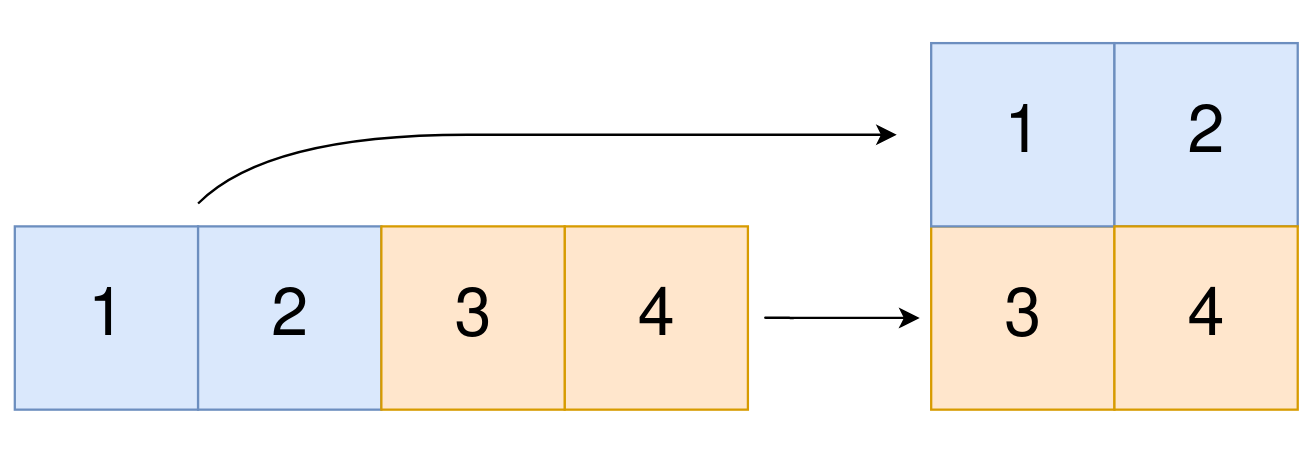2022. Convert 1 D Array Into 2 D Array

# 2022. Convert 1D Array Into 2D Array#

## 题目 #

You are given a 0-indexed 1-dimensional (1D) integer array `original`, and two integers, `m` and `n`. You are tasked with creating a 2-dimensional (2D) array with `m` rows and `n` columns using all the elements from `original`.

The elements from indices `0` to `n - 1` (inclusive) of `original` should form the first row of the constructed 2D array, the elements from indices `n` to `2 * n - 1` (inclusive) should form the second row of the constructed 2D array, and so on.

Return an `m x n` 2D array constructed according to the above procedure, or an empty 2D array if it is impossible.

Example 1:``````Input: original = [1,2,3,4], m = 2, n = 2
Output: [[1,2],[3,4]]
Explanation: The constructed 2D array should contain 2 rows and 2 columns.
The first group of n=2 elements in original, [1,2], becomes the first row in the constructed 2D array.
The second group of n=2 elements in original, [3,4], becomes the second row in the constructed 2D array.

``````

Example 2:

``````Input: original = [1,2,3], m = 1, n = 3
Output: [[1,2,3]]
Explanation: The constructed 2D array should contain 1 row and 3 columns.
Put all three elements in original into the first row of the constructed 2D array.

``````

Example 3:

``````Input: original = [1,2], m = 1, n = 1
Output: []
Explanation: There are 2 elements in original.
It is impossible to fit 2 elements in a 1x1 2D array, so return an empty 2D array.

``````

Constraints:

• `1 <= original.length <= 5 * 104`
• `1 <= original[i] <= 105`
• `1 <= m, n <= 4 * 104`

## 题目大意 #

original 中下标从 0 到 n - 1 （都 包含 ）的元素构成二维数组的第一行，下标从 n 到 2 * n - 1 （都 包含 ）的元素构成二维数组的第二行，依此类推。

## 解题思路 #

• 简单题。从一维数组 original 中依次取出每行 n 个元素，顺序放到 m 行中。此题中，如果 m*n 大于或者小于 original 的长度，都输出空数组。

## 代码 #

``````package leetcode

func construct2DArray(original []int, m int, n int) [][]int {
if m*n != len(original) {
return [][]int{}
}
res := make([][]int, m)
for i := 0; i < m; i++ {
res[i] = original[n*i : n*(i+1)]
}
return res
}
``````Apr 8, 2023Edit this page